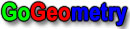#Geometry Problem 1317: Triangle, Excircle, Chord, Tangent, Midpoint, Arc, Sum of two Segments, Congruence.

 < PREVIOUS PROBLEM  |  NEXT PROBLEM > The figure shows a triangle ABC with the excircle O corresponding to BC (D, E, and F are tangency points). M is the midpoint of arc DE. BM and CM cut chord DE at G and H, respectively. Prove that DG + HE = GH.See also: Sketch of problem 1317 using mobile apps

 Home | Geometry | Problems | All Problems | Open Problems | Visual Index | 1311-1320 | Triangle | Circle | Excircle | Tangent line | Midpoint | Congruence | View or Post a solution | by Antonio Gutierrez